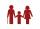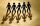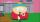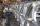# Quadratic equation + reason - math problems

#### Number of problems found: 48

• Right triangle eq2Find the lengths of the sides and the angles in the right triangle. Given area S = 210 and perimeter o = 70.
• Young mathematicianOne young mathematician was bored again. He found that the average age of people in the room where the seminar equals to its count. Then his 29-year-old brother entered this room. Even then, the average age of all present was the same as the count of peop
• Find twoFind two consecutive natural numbers whose product is 1 larger than their sum. Searched numbers expressed by a fraction whose numerator is the difference between these numbers and the denominator is their sum.
• Digit sumThe digit sum of the two-digit number is nine. When we turn figures and multiply by the original two-digit number, we get the number 2430. What is the original two-digit number?
• Wagons and cranesSeveral of the same cranes unloaded 96 wagons. If there were 2 more cranes there would be less 8 wagons for each crane. How many cranes were here?
• Average ageThe average age of all people at the celebration was equal to the number of people present. After the departure of one person who was 29 years old, average age was again equal to the number present. How many people were originally to celebrate?
• VariableFind variable P: PP plus P x P plus P = 160
• Two trucksTwo trucks left cities A and B against each other and met after an hour. The first car came to B 27 minutes later than the second car to A. Calculate the car speed if the distance between cities A, B is 90 km.
• Rectangle diagonalsIt is given a rectangle with an area of 24 cm2 a circumference of 20 cm. The length of one side is 2 cm larger than the length of the second side. Calculate the length of the diagonal. Length and width are yet expressed in natural numbers.
• Four integersFnd four consecutive integers so that the product of the first two is 70 times smaller than the product of the next two.
• Difference of two numberThe difference between the two numbers is 20. They are positive integers greater than zero. The first number raised to one-half equals the second number. Determine the two numbers.
• Triangle ABCTriangle ABC has side lengths m-1, m-2, m-3. What has to be m to be triangle a) rectangular b) acute-angled?
• RectangleThe perimeter of the rectangle is 22 cm and content area 30 cm2. Determine its dimensions, if the length of the sides of the rectangle in centimeters is expressed by integers.
• FriendsSome friends had to collect the sum 72 EUR equally. If the three refused their part, others would have to give each 4 euros more. How many are friends?
• Do you solve this?Determine area S of rectangle and length of its sides if its perimeter is 102 cm.
• QuizQ2The square of the first number is equal to three-fifths of the second number. Determine both numbers if you know that the second number is 5 times greater than the first, and neither of numbers is not equal to zero.
• AutomakerThe automaker now produces daily 4 new cars more than last year so the production of 360 cars will save just one full working day. How many working days to produce 360 vehicles needed last year?
• CircleCircle is given by centre on S[-7; 10] and maximum chord 13 long. How many intersect points have circle with the coordinate axes?
• RootThe root of the equation (x-19)2 -10 = x2 -11x is (equal or greater or less than zero)? ...
• GardenThe area of a square garden is 2/9 of triangle garden with sides 160 m, 100 m, and 100 m. How many meters of fencing need to fence a square garden?

Do you have an exciting math question or word problem that you can't solve? Ask a question or post a math problem, and we can try to solve it.

We will send a solution to your e-mail address. Solved examples are also published here. Please enter the e-mail correctly and check whether you don't have a full mailbox.

Looking for help with calculating roots of a quadratic equation? Quadratic Equations Problems. Reason - math problems.